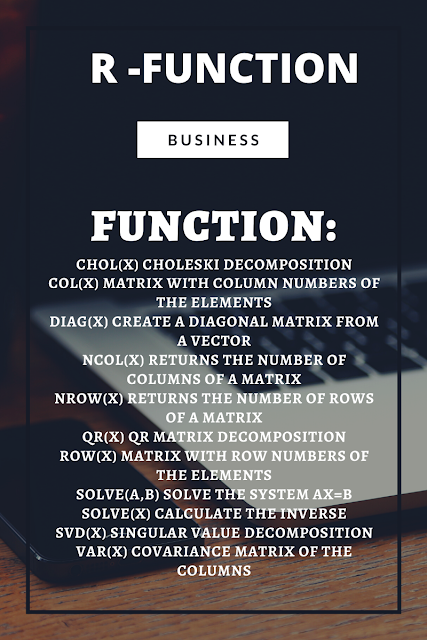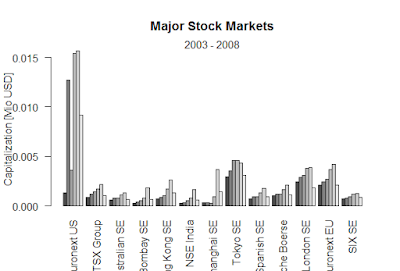Showing posts with label Function. Show all posts
Showing posts with label Function. Show all posts

## Function:

chol(x) Choleski decomposition col(x) Matrix with column numbers of the elements diag(x) Create a diagonal matrix from a vector ncol(x) Returns the number of columns of a matrix nrow(x) Returns the number of rows of a matrix qr(x) QR matrix decomposition row(x) Matrix with row numbers of the elements solve(A,b) Solve the system Ax=b solve(x) Calculate the inverse svd(x) Singular value decomposition var(x) Covariance matrix of the columnsFUNCTION

### Black-Scholes formula-R

Black-Scholes formula-R > BlackScholes <- function(TypeFlag = c("c", "p"), S, X, Time, r, b, sigma) { TypeFla...